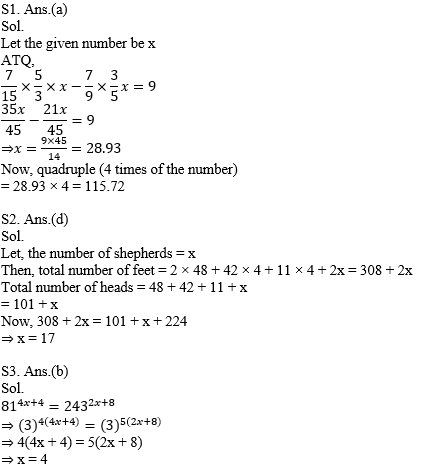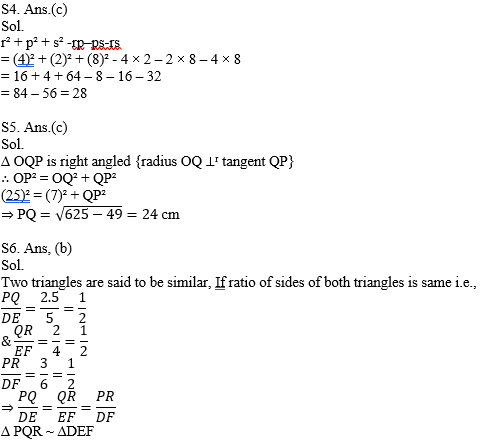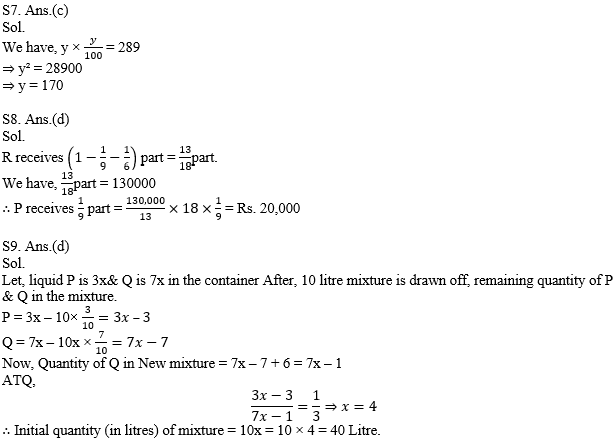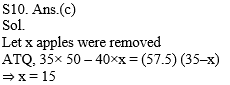### CTET/ UPTET Exam Practice Mathematics Miscellaneous Questions

Dear Students!!! There is most general as well as a scoring section in all the competitive entrance examinations in the teaching field i.e "Mathematics".Because in this section only one thing is work i.e your accuracy and that could be nourished with the daily practice.With proper system, Study Notes, Quizzes, Vocabulary one can quiet his/her nerves and exceed expectations in the blink of an eye. So, for this, we are providing you the daily quiz for all teaching exams i.e CTET Exam 2019DSSSB ,KVS,STET Exam.

Q1. 7/15 of 5/3 of a number is greater than 7/9 of 3/5 of the same number by 9. Find the quadruple of that number.

(a) 115.72
(b)114.72
(c) 113.72
(d)112.72

Q2. In a farm, in addition to 48 hens there are 42 sheep and 11 oxen with some shepherds. If the total number of feet be 224 more than the number of heads, find the number of shepherd.

(a) 11
(b)13
(c) 15
(d)17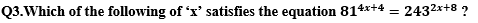(a) 3
(b)4
(c) 5
(d)6

54. What will be the value of r² + p² + s² – rp – ps – rs when r = 4, p = 2 and s = 8 ?

(a) 8
(b)18
(c) 28
(d)38

Q5. Calculate the length (in cm) of PQ, if the radius of the circle is 7 cm and the line through the centre O meets the tangent at point Q such that OP = 25 cm.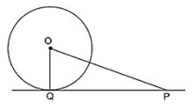(a) 20
(b)22
(c) 24
(d)26

Q6. Consider the following figure shown below and choose which of the following equation is correct about the similarity of both triangles ?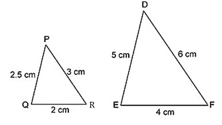(a) ∆PQR ~ ∆EFD
(b)∆PQR ~ ∆DEF
(c) ∆PQR ~ ∆FDE
(d)∆RQP ~ ∆DFE

Q7. If y% of y is 289, then what is the value of y?

(a) 149
(b)160
(c) 170
(d)173

Q8. P, Q and R are partners in a company. In any year P receives 1/9 part of profit, Q receives 1/6 part of profit and R receives Rs 130000. How much amount (in Rs) will P get as profit?

(a) 40000
(b)36000
(c) 18000
(d)20000

Q9. In a container, two types of liquids P and Q are in the ratio of 3 : 7. If 10 litres mixture is drawn off from the container and filled with 6 litres of liquid Q, then the ratio of P and Q becomes 1 : 3. What was the original quantity (in litres) of mixture?

(a) 34
(b)36
(c) 30
(d)40

Q10. The average weight of 35 apples is 50 grams. If some apples of weight 40 grams each were removed, then average weight becomes 57.5 grams. How many apples of weight 40 grams were removed?

(a) 5
(b)10
(c) 15
(d)20

Solutions